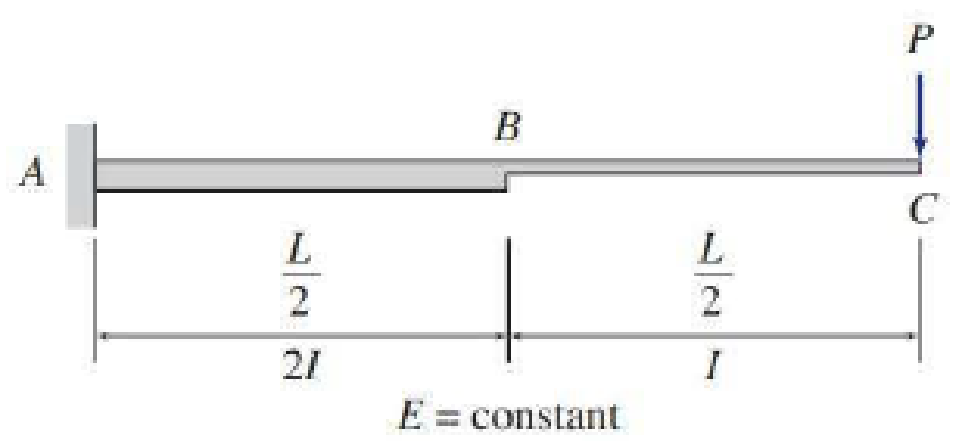# 7.28 through 7.30 Determine the deflection at point C of the beam shown in Fig. P7.24–P7.26 by the virtual work method. Use the graphical procedure (Table 7.6) to evaluate the virtual work integrals. FIG. P7.24, P7.28, P7.59

#### Solutions

Chapter
Section
Chapter 7, Problem 28P
Textbook Problem
35 views

## 7.28 through 7.30 Determine the deflection at point C of the beam shown in Fig. P7.24–P7.26 by the virtual work method. Use the graphical procedure (Table 7.6) to evaluate the virtual work integrals.FIG. P7.24, P7.28, P7.59

To determine

Find the deflection at point C of the beam using virtual work method.

### Explanation of Solution

Given information:

The beam is given in the Figure.

The value of E is constant.

Apply the sign conventions for calculating reactions, forces and moments using the three equations of equilibrium as shown below.

• For summation of forces along x-direction is equal to zero (Fx=0), consider the forces acting towards right side as positive (+) and the forces acting towards left side as negative ().
• For summation of forces along y-direction is equal to zero (Fy=0), consider the upward force as positive (+) and the downward force as negative ().
• For summation of moment about a point is equal to zero (Matapoint=0), consider the clockwise moment as negative and the counter clockwise moment as positive.

Calculation:

Consider the real system.

Sketch the real system of the beam as shown in Figure 1.

Refer Figure 1 to find the reaction and moment at A.

Summation of forces along y-direction is equal to 0.

+Fy=0AyP=0Ay=P

Summation of moments about A is equal to 0.

MA=0MAP(L)=0MA=PL

Find the moment at point B:

MB=P×L2=PL2

Sketch the moment diagram of the real system M as shown in Figure 2.

Consider the virtual system.

Remove all the real loads and apply unit load where the point to find the deflection.

Let the bending moment due to virtual load be Mv.

Sketch the virtual system of the beam with unit load at point B as shown in Figure 3.

Refer Figure 1 to find the reaction and moment at A.

Summation of forces along y-direction is equal to 0.

+Fy=0Ay1=0Ay=1

Summation of moments about A is equal to 0.

MA=0MA1(L)=0MA=L

Find the moment at point B:

MB=1×L2=L2

Sketch the moment diagram of the virtual system Mv as shown in Figure 4.

Find the deflection at C using the virtual work expression:

1(ΔC)=0LMvMEIdx (1)

Substitute 2I for span AB and I for span BC.

Rearrange Equation (1) for the limits 0L/2 and L/2L as follows.

ΔC=1EI[120L/2(MvM)dx+L/2L(MvM)dx] (2)

Refer Table 7.6, “Integrals 0LMvMdx for moment diagrams of simple geometric shapes” in the textbook.

For the moment diagram in Figure 2 and Figure 4, the value of 0L/2(MvM)dx and L/2L(MvM)dx is 16(Mv1(2M1+M2)+Mv2(M1+2M2))L and 13Mv1M1L

### Still sussing out bartleby?

Check out a sample textbook solution.

See a sample solution

#### The Solution to Your Study Problems

Bartleby provides explanations to thousands of textbook problems written by our experts, many with advanced degrees!

Get Started

Find more solutions based on key concepts
Given that the three bars shown in the accompanying figure are made of the same material, comparing bar (a) to ...

Engineering Fundamentals: An Introduction to Engineering (MindTap Course List)

Content Management System Updates You are attempting to update your companys website using a content management...

Enhanced Discovering Computers 2017 (Shelly Cashman Series) (MindTap Course List)

The force system shown can be replaced with a single, equivalent couple CR. Determine CR.

International Edition---engineering Mechanics: Statics, 4th Edition

If your motherboard supports ECC DDR3 memory, can you substitute non-ECC DDR3 memory?

A+ Guide to Hardware (Standalone Book) (MindTap Course List)

Describe the three classifications of burns.

Welding: Principles and Applications (MindTap Course List)